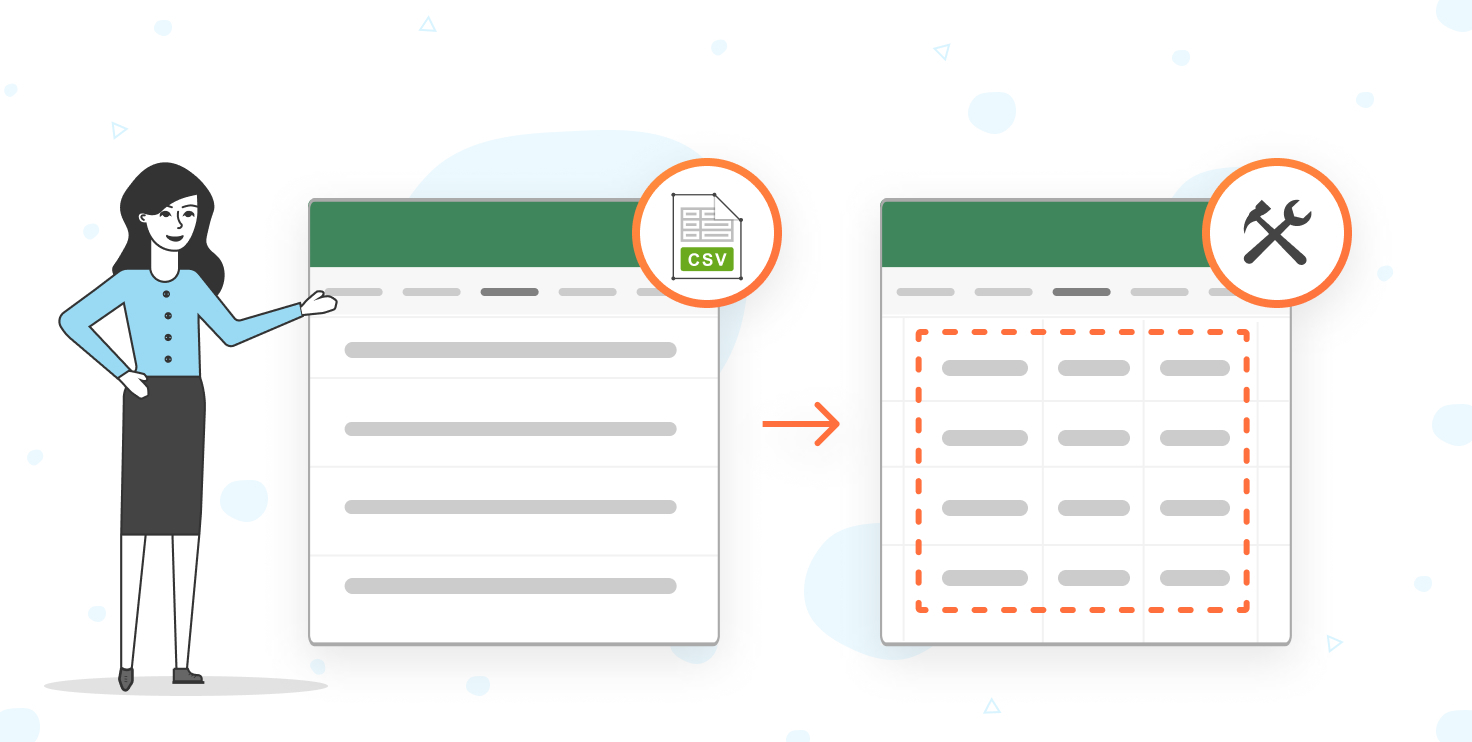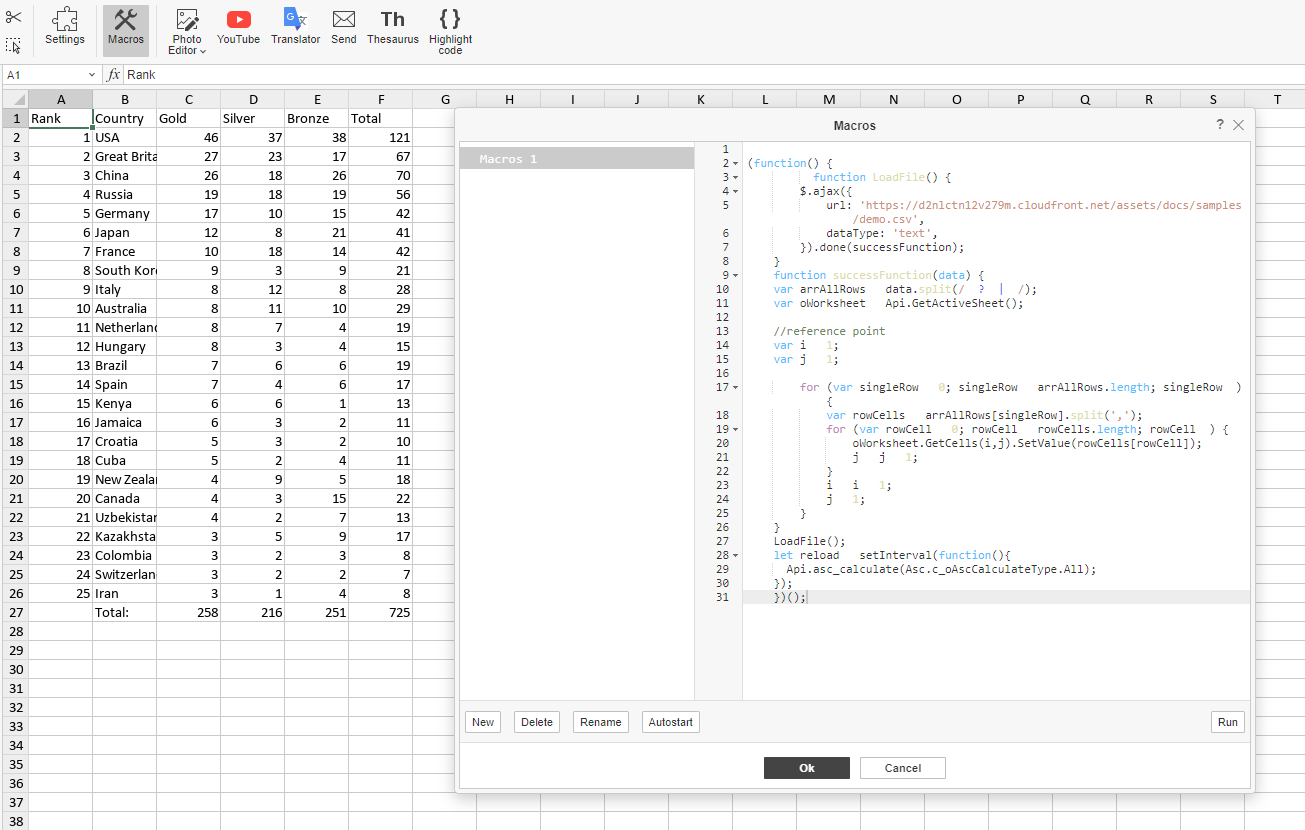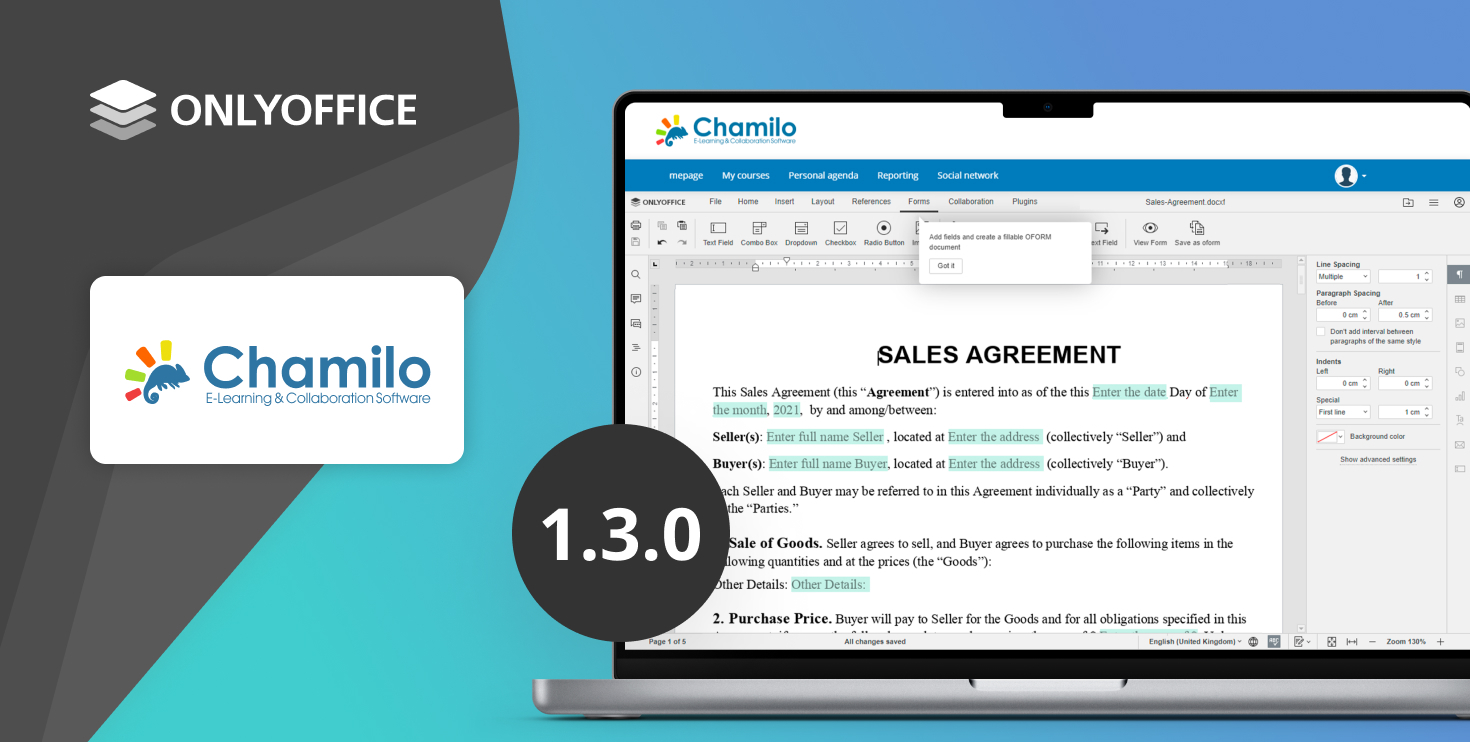# 使用 ONLYOFFICE 宏向电子表格中导入 CSV 与文本数据

2022日10月18日## 构建宏

``````      function LoadFile() {
\$.ajax({
dataType: 'text',
}).done(successFunction);
}
}
``````

`````` function successFunction(data) {
var arrAllRows = data.split(/\r?\n|\r/);
``````

``````var oWorksheet = Api.GetActiveSheet();
``````

``````   for (var singleRow = 0; singleRow < arrAllRows.length; singleRow++) {
var rowCells = arrAllRows[singleRow].split(',');
}
``````

`````` for (var rowCell = 0; rowCell < rowCells.length; rowCell++) {
oWorksheet.GetCells(i,j).SetValue(rowCells[rowCell]);
j = j + 1;
}
i = i + 1;
j = 1;
}
}
``````

``````  let reload = setInterval(function(){
Api.asc_calculate(Asc.c_oAscCalculateType.All);
});
``````

``````(function() {
\$.ajax({
dataType: 'text',
}).done(successFunction);
}
function successFunction(data) {
var arrAllRows = data.split(/\r?\n|\r/);
var oWorksheet = Api.GetActiveSheet();

//reference point
var i = 1;
var j = 1;

for (var singleRow = 0; singleRow < arrAllRows.length; singleRow++) {
var rowCells = arrAllRows[singleRow].split(',');
for (var rowCell = 0; rowCell < rowCells.length; rowCell++) {
oWorksheet.GetCells(i,j).SetValue(rowCells[rowCell]);
j = j + 1;
}
i = i + 1;
j = 1;
}
}
Api.asc_calculate(Asc.c_oAscCalculateType.All);
});
})();
``````### 最新博文## 用于 Chamilo 的 ONLYOFFICE 连接器 v1.3.0：演示服务器、JWT 标头配置、内部请求的地址等更新

2023日11月03日作者: Mona